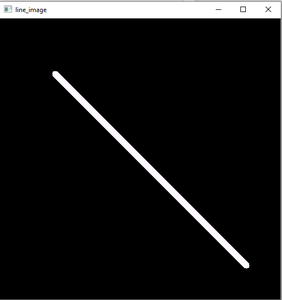# Python OpenCV | cv2.line() method

• Last Updated : 10 Jun, 2022

OpenCV-Python is a library of Python bindings designed to solve computer vision problems.cv2.line() method is used to draw a line on any image.

Syntax:

`cv2.line(image, start_point, end_point, color, thickness) `

Parameters: image: It is the image on which line is to be drawn.

• start_point: It is the starting coordinates of the line. The coordinates are represented as tuples of two values i.e. (X coordinate value, Y coordinate value).
• end_point: It is the ending coordinates of the line. The coordinates are represented as tuples of two values i.e. (X coordinate value, Y coordinate value).
• color: It is the color of the line to be drawn. For RGB, we pass a tuple. eg: (255, 0, 0) for blue color.
• thickness: It is the thickness of the line in px

Return Value: It returns an image.

### Implementation:

The image used for examples n1 and 2 is as follows:Example #1:

## Python3

 `# Python program to explain cv2.line() method`` ` `# importing cv2``import` `cv2`` ` `# path``path ``=` `r``'C:\Users\Rajnish\Desktop\geeksforgeeks\geeks.png'`` ` `# Reading an image in default mode``image ``=` `cv2.imread(path)`` ` `# Window name in which image is displayed``window_name ``=` `'Image'` `# Start coordinate, here (0, 0)``# represents the top left corner of image``start_point ``=` `(``0``, ``0``)` `# End coordinate, here (250, 250)``# represents the bottom right corner of image``end_point ``=` `(``250``, ``250``)` `# Green color in BGR``color ``=` `(``0``, ``255``, ``0``)` `# Line thickness of 9 px``thickness ``=` `9` `# Using cv2.line() method``# Draw a diagonal green line with thickness of 9 px``image ``=` `cv2.line(image, start_point, end_point, color, thickness)` `# Displaying the image``cv2.imshow(window_name, image)`

Output:Example #2:

## Python3

 `# Python program to explain cv2.line() method`` ` `# importing cv2``import` `cv2`` ` `# path``path ``=` `r``'C:\Users\Rajnish\Desktop\geeksforgeeks\geeks.png'`` ` `# Reading an image in grayscale mode``image ``=` `cv2.imread(path, ``0``)`` ` `# Window name in which image is displayed``window_name ``=` `'Image'` `# Start coordinate, here (225, 0)``# represents the top right corner of image``start_point ``=` `(``225``, ``0``)` `# End coordinate, here (0, 225)``# represents the bottom left corner of image``end_point ``=` `(``0``, ``225``)` `# Black color in BGR``color ``=` `(``0``, ``0``, ``0``)` `# Line thickness of 5 px``thickness ``=` `5` `# Using cv2.line() method``# Draw a diagonal black line with thickness of 5 px``image ``=` `cv2.line(image, start_point, end_point, color, thickness)` `# Displaying the image``cv2.imshow(window_name, image)`

Output:Example 3: Drawing a line on black screen using numpy library:

## Python3

 `import` `numpy as np``import` `cv2``# Creating a black screen image using nupy.zeros function``Img ``=` `np.zeros((``512``, ``512``, ``3``), dtype``=``'uint8'``)``# Start coordinate, here (100, 100). It represents the top left corner of image``start_point ``=` `(``100``, ``100``)``# End coordinate, here (450, 450). It represents the bottom right corner of the image according to resolution``end_point ``=` `(``450``, ``450``)``# White color in BGR``color ``=` `(``255``, ``250``, ``255``)``# Line thickness of 9 px``thickness ``=` `9``# Using cv2.line() method to draw a diagonal green line with thickness of 9 px``image ``=` `cv2.line(Img, start_point, end_point, color, thickness)``# Display the image``cv2.imshow(``'Drawing_Line'``, image)``cv2.waitKey(``0``)``cv2.destroyAllWindows()`

Output:Black Screen created using numpy and drawing line using cv2.line() function

My Personal Notes arrow_drop_up